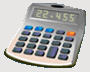DosageHelp.com Helping Nursing Students Prepare for Medication Exams By Explaining Dosage Calculations
The following is an overview of the steps you should take to complete each dosage problem. The rest of this tutorial will explain how to perform each of these steps.

# Dosage Question Steps

1. Determine problem type:
2. Identify what is the value of each variable in formula.
3. Make units consistent as necessary.
4. Fill in values for formula and solve the problem.

Home Dosage Question Steps Unit Conversion Mass for Mass Mass/Liquid For Liquid IV Terms & Abbreviations Amount in IV Fluid Volume/Time - IV mL Rate Volume/Time - IV Drop Rate Fluid Maintenance Dosage By Weight Mass/Time - IV mL Rate Practice Questions Question of the Day Tell a Friend Print a Flyer